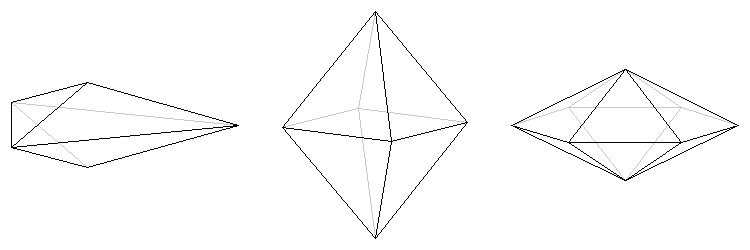Equable Isosceles Dipyramid

EQUABLE ISOSCELES DIPYRAMID

Balmoral Software

Solutions: 3
(6, 8 or 12 faces)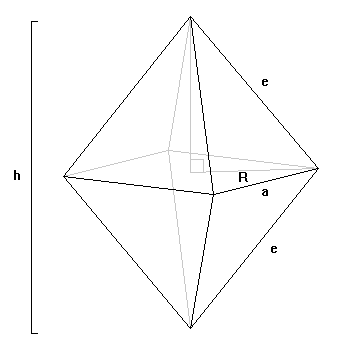A dipyramid is created from two identical right pyramids, each of which has a base that is a regular polygon of n sides of length a, joined base-to-base. The height of the entire dipyramid is h. The area of the regular polygon is
Bn = (n/4)a2cot(180°/n)
The volume of the dipyramid is twice that of the pyramid:
 V = 2(1/3)(h/2)Bn = (n/12)a2hcot(180°/n) 
and is assumed to be an integer by convention. The 2n faces of the dipyramid are identical isosceles triangles, each of which has a base of length a and two sides of length e, where
e2 = (h/2)2 + R2
and R is the circumradius of the regular polygon:
 R = (a/2)csc(180°/n) 
The area of each triangle then is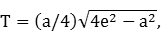which is also assumed to be an integer by convention. By equability,
 V = 2nT = S, 
which reduces to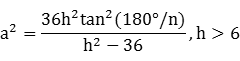Substituting  in  and , we have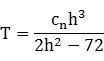where the real number cn is defined as
 cn = 3tan(180°/n) 
Some values of cn are: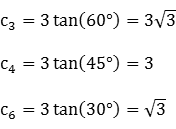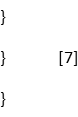For h > 6, T has a minimum value of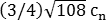at h =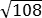, and two real solutions for h exist for each integer value of T above this minimum. Therefore, there are infinitely-many equable isosceles dipyramids. We henceforth restrict the analysis to the more distinctive cases where cn is the square root of an integer, which occurs only when n = 3, 4 or 6. We can see from  and  that T can be an integer only when h is an integer (n = 4), or h is an integer multiple of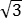(n = 3 or 6).

Case n = 4

In the first case, we have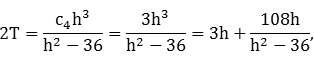so the fraction on the right is a positive integer, and therefore its numerator is at least as large as its denominator:
108h ≥ h2 - 36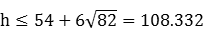We can iterate  over the range 7 ≤ h ≤ 108 to find all solutions for which 2T is an even integer; the single result is
h = 12, T = 24

Case n = 3

Now let h =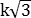for some positive integer k. For n = 3, we have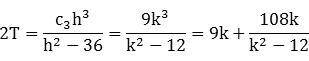As before,
108k ≥ k2 - 12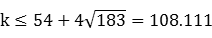If h > 6, then k >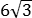= 3.464, so we iterate over the range 4 ≤ k ≤ 108 to find the solution
k = 4, h =, T = 72

Case n = 6

Similarly, we have for n = 6: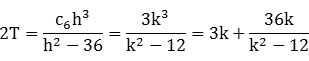36k ≥ k2 - 12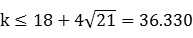We can then iterate over the range 4 ≤ k ≤ 36 to find the solution
k = 4, h =, T = 24
It follows from  and  that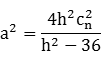and from  that
R2 = (a2/4)(1 + 9/cn2)

Summary

The three equable solutions are:

ncnhaReTV = S
3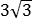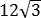12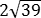72432
4312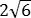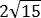24192
624288
Both the equable octahedron and the equable dodecahedron have identically-sized faces.